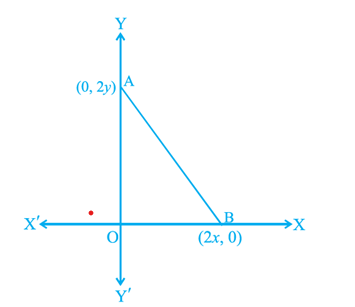Filter By

## All Questions

#### If the points A (1, 2), O (0, 0) and C (a, b) are collinear, then(A) a = b(B) a = 2b(C) 2a = b(D) a = –b

If points A(1, 2), O(0, 0),C (a, b) are collinear then area of triangle

formed by these points must be zero.

Hence, option C is correct.

#### If the distance between the points (4, p) and (1, 0) is 5, then the value of p is(A) 4 only(B) ± 4(C) – 4 only(D) 0

Points are A(4, p) and B(1, 0)

Distance

Here

Using distance formula

Hence, option B is correct.

## Crack CUET with india's "Best Teachers"

• HD Video Lectures
• Unlimited Mock Tests
• Faculty Support#### The area of a triangle with vertices (a, b + c), (b, c + a) and (c, a + b) is(A) (a+b+c)2(B) 0(C)( a + b + c)(D) abc

Vertices are (a, b + c), (b, c + a), (c, a + b)

Here

We know that

Hence, option B is correct.

#### A line intersects the y-axis and x-axis at the points P and Q, respectively. If(2, –5) is the mid-point of PQ, then the coordinates of P and Q are respectively(A) (0, – 5) and (2, 0)(B) (0, 10) and (– 4, 0)(C) (0, 4) and (– 10, 0)(D) (0, – 10) and (4, 0)

Point

Hence, option D is correct.

## Crack NEET with "AI Coach"

• HD Video Lectures
• Unlimited Mock Tests
• Faculty Support#### A circle drawn with origin as the Centre passes through(13/2, 0) . The point which does not lie in the interior of the circle is :

A) Distance of the point (-3/4, 1) from (0,0) is

The distance  1.25<6.5 .  so the point (-3/4, 1)  is lie
B) Distance point (2, 7/3) from (0,0) is

So the point is lie
C) Distance point (5,-1/2) from (0,0) is

So the point is lie in the interior of the circle
D)
The circle passes through (13/2, 0) having a centre (0,0)

Hence, point D is the correct answers.

#### The coordinates of the point whichis equidistant from the three verticesof the ΔAOB as shown in the figure isIn the given figure, it is clear that DAOB is a right-angle triangle.

And in a right-angle triangle, the mid-point of the hypotenuse is equidistant from the three vertices. Thus, co-ordinates must be mid-point of AB

Now find mid-point of AB using mid-point formula

Hence, option A is correct.

## Crack JEE Main with "AI Coach"

• HD Video Lectures
• Unlimited Mock Tests
• Faculty Support#### The perpendicular bisector of the line segment joining the points A (1, 5) andB (4, 6) cuts the y-axis at(A) (0, 13)(B) (0, –13)(C) (0, 12)(D) (13, 0)

Squaring both sides we get

Hence, point P is (0, 13)

Therefore, option A is correct.

#### If P(a/3 , 4) is the mid-point of the line segment joining the points Q (– 6, 5) and R (– 2, 3), then the value of a is(A) – 4(B) – 12(C) 12(D) – 6

By using mid-point formula

Compare x co-ordinate

Hence, option B is correct.

## Crack CUET with india's "Best Teachers"

• HD Video Lectures
• Unlimited Mock Tests
• Faculty Support#### If the point P (2, 1) lies on the line segment joining points A (4, 2) and B (8, 4),then

Let P divide  A B  in ratio  k: 1

By using section formula
Here,

Compare x co-ordinate

#### The fourth vertex D of a parallelogram ABCD whose three vertices areA (–2, 3), B (6, 7) and C (8, 3) is(A) (0, 1)(B) (0, –1)(C) (–1, 0)(D) (- 2 , 0)

Let D(x, y)

A(–2, 3), B(6, 7), C(8, 3) (given)

We know that in parallelogram diagonals are equal

mid-point of AC = mid-point of BD

option B is correct.